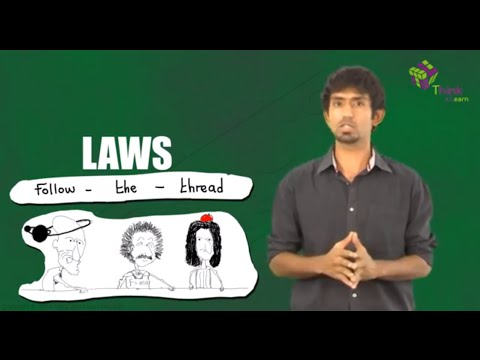# Types of Moment of Inertia

There are three types of the moment of inertia:

• Mass moment of inertia
• Area moment of inertia
• Polar moment of inertia

Mass moment of inertia

Mass moment of inertia is defined as the resistance offered by the body to rotate due to its own mass. Examples of mass moment of inertia is a ceiling fan, impeller, and flywheel. Mass moment of inertia for objects must be less to reduce power consumption. Inertia for such bodies is taken about the axis of rotation.

Area moment of inertia

Area moment of inertia is defined as the resistance offered by an object either to bend or to deflect. Here, inertia is independent of mass and is dependent on the area of the object. The beam is an example of an area moment of inertia.

Polar moment of inertia

The resistance offered by the object to the torsion is known as the polar moment of inertia. The polar moment is different from the mass moment of inertia, as the twist is different from rotation. The shaft is an example of a polar moment of inertia.### Refer more(35)(2)